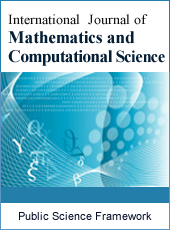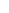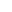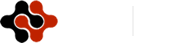International Journal of Mathematics and Computational Science
Articles Information
International Journal of Mathematics and Computational Science, Vol.1, No.6, Dec. 2015, Pub. Date: Dec. 6, 2015
Asymptotic Analytical Solutions of an Electrostatically Actuated Microbeam Base on Homotopy Analysis Method
 Y. H. Qian, College of Mathematics, Physics and Information Engineering, Zhejiang Normal University, Jinhua, Zhejiang, P. R. China;Department of Mathematics, Shawnee State University, Portsmouth, OH, USA.
 W. K. Liu, College of Mathematics, Physics and Information Engineering, Zhejiang Normal University, Jinhua, Zhejiang, P. R. China.
 L. Shen, College of Mathematics, Physics and Information Engineering, Zhejiang Normal University, Jinhua, Zhejiang, P. R. China.
 S. P. Chen, Department of Mathematics, Xiamen University of Technology, Xiamen, P. R. China.
Presented herein is an analytical approach based on homotopy analysis method (HAM) used to deal with the seventh-order Duffing type problem with high-order nonlinearity. Such a problem corresponds to the large-amplitude vibration of an electrostatically actuated microbeam. Unlike tradition HAM, the convergence-control auxiliary parameters are introduced in the present approximation to improve the accuracy. To verify the efficient of present approach, illustrative examples are provided and compared between the results obtained by analytical and numerical method.
Homotopy Analysis Method, Electrostatically Actuated Microbeam, Convergence-Control Parameter
 Lyshevski S. E., MEMS and NEMS: Systems, Devices and Structures. Boca Raton, CRC Press, 2002.
 Qian Y. H., Ren D. X., Lai S. K. and Chen S. M.. Analytical approximations to nonlinear vibration of an electrostatically actuated microbeam, Communications in Nonlinear Science and Numerical Simulation, Vol. 17, pp. 1947–1955, 2012.
 Ghanbari M., Yazdapanah M. J.. Delay compensation of tilt sensors based on MEMS accelerometer using data fusion technique, IEEE Sensors Journal, Vol. 15, pp. 1959–1966, 2015.
 Sabri M. F. M., Ono T., Said S. M., Kawai Y. and Esashi M.. Fabrication and characterization of microstacked PZT actuator for MEMS applications, Journal of Microelectromechanical Systems, Vol. 24, pp. 80–90, 2015.
 Vahabisani N., Daneshmand M.. Monolithic millimeter-wave MENS waveguide switch, IEEE Transactions on Microwave Theory and Techniques, Vol. 63, pp. 340–351, 2015.
 Xi Z. W., Cao Y., Yu P. X., Nie W. R. And Wang J.. The simulation and visual test contact process of a MEMS inertial switch with flexible electrodes, Microsyst Technol, DOI 10.1007/s00542-2509-4, 2015.
 Fu Y. M., Zhang J. and Wan L. J.. Application of the energy balance method to a nonlinear oscillator arising in the microelectromechanical system (MEMS), Current Applied Physics , Vol. 11, pp. 482-485, 2011.
 Pu X., Li W. H. and Zhou Z. F.. Electrothermal-driven gap adjustable MEMS comb structure: modeling and simulation of the equivalent circuit macromodel, Microsyst Technol, Vol. 20, pp.1205-1212, 2014.
 He J. H.. Variaional iteration method some recent results and new interpretations, Journal of Computational and Applied Mathematics, Vol.207, pp. 3-17, 2007.
 He J. H., Wu X. H.. Construction of solitary solution compaction like solution by variational iteration method, Chaos Solitions & Fractals, Vol. 29, pp. 103-108, 2006.
 Xu L..Variational principles for coupled nonliear Schrodinger equations, Physics Letters A, Vol. 359, pp. 627-29, 2006.
 Rafei M, Ganji D. D, Daniali H. and Pashaei H.. The variational iteration method for nonlinear oscillators with discontinuities, Journal of Sound and Vibration, Vol.305, pp. 614-620, 2007.
 Cheung Y. K., Chen S. H. and Lau S. L.. A modified Lindstedt–Poincaré method for certain nonlinear oscillators, International Journal of Non-linear Mechanics, Vol.26, pp. 367-378,1991.
 Senator R. R., Bapat C. N.. A perturbation technique that works even when the non-linearity is not small, Journal of Sound and Vibration, Vol. 164, pp. 1-27, 1993.
 Amore P., Aranda A.. Improved Lindstedt–Poincaré method for the solution of nonlinear problems, Journal of Sound and Vibration, Vol. 283, pp. 1115-1136, 2005.
 Pušenjak R. R.. Extended Lindstedt–Poincare method for non-stationary resonances of dynamical systems with cubic nonlinearities, Journal of Sound and Vibration, Vol.314, pp. 194-216, 2008.
 Wang S. Q., He J. H.. Nonlinear oscillator with discontinuity by parameter expansion method, Chaos Solitons & Fractals, Vol. 35, pp. 688-691, 2008.
 Noor M. A., Mohyud-Din S. T.. Parameter-expansion techniques for strongly nonlinear oscillators, International Journal of Nonlinear Sciences and Numerical Simulation, Vol. 10, pp. 581-583, 2009.
 Huseyin K. L., Lin R.. An intrinsic multiple-scale harmonic balance method for non-linear vibration and bifurcation problems, International Journal of Non-linear Mechanics, Vol. 26, pp. 727-740, 1991.
 Seeling F. F.. Unrestricted harmonic balance II. Application to stiff ordinary differential equations in enzyme catalysis, Journal of Mathematical Biology, Vol. 12, pp.187-198, 1981.
 Summers J. L., Savage M. D.. Two timescale harmonic-balance. I. Application to autonomous one-dimensional nonlinear oscillators, Philos. Trans. R. Soc. A: Math. Phys. Eng. Sci, Vol. 340, pp. 473–501, 1992.
 Wu B. S., Li P. S.. A method for obtaining approximate analytic periods for a class of nonlinear oscillators, Meccanica, Vol. 36, pp. 167–176, 2001.
 He J. H.. Maxemin approach to nonlinear oscillators, International Journal of Nonlinear Sciences and Numerical Simulation, Vol. 9, pp. 207-210, 2008.
 Zeng D. Q.. Nonlinear oscillator with discontinuity by the max-min approach, Chaos Solitons & Fractals, Vol. 42, pp. 2885-2889, 2009.
 Zeng D. Q., Lee Y. Y.. Analysis of strongly nonlinear oscillator using the maxemin approach, International Journal of Nonlinear Sciences and Numerical Simulation, Vol. 10, pp. 1361-1368, 2009.
 He J. H.. Preliminary report on the energy balance for nonlinear oscillations, Mechanics Research Communication, Vol.29, pp. 107-11, 2002.
 He J. H.. Some asymptotic methods for strongly nonlinear equation, International Journal of Modern Physics B, Vol. 20, pp. 1141-99, 2006.
 Liao S. J.. The proposed homotopy analysis techniques for the solution of nonlinear problems, Ph.D. dissertation, Shanghai Jiao Tong University, China, 1992.
 Liao S. J.. An approximate solution technique not depending on small parameters: a special example, International Journal of Non-linear Mechanics, Vol. 30, pp. 371-380, 1995.
 Liao S. J.. Beyond Perturbation: Introduction to the Homotopy Analysis Method, Chapman & Hall, Boca Raton, 2003.
 Liao S. J.. An optimal homotopy-analysis approach for strongly nonlinear differential equations, Communications in Nonlinear Science and Numerical Simulation, Vol. 15, pp. 2003-2016, 2010.
 Niu Z, Wang C.. A one-step optimal homotopy analysis method for nonlinear differential equations, Communications in Nonlinear Science and Numerical Simulation, Vol15, pp. 2026-2036, 2010.
 Qian Y. H, Lai S. K., Zhang W. and Xiang Y.. Study on asymptotic analytical solutions using HAM for strongly nonlinear vibrations of a restrained cantilever beam with an intermediate lumped mass, Numerical Algorithms, Vol. 58, pp. 293-314, 2011.
 Abbasbandy•M. Jalili S.. Determination of optimal convergence-control parameter value in homotopy analysis method, Numerical Algorithms, Vol. 64, pp. 593-605, 2013.
 Mallory K., Van Gorder R A., Optimal homotopy analysis and control of error for solutions to the non-local Whitham equation, Numerical Algorithms, Vol. 66, pp. 843-863, 2014.
 Pelesko J. A., Bernstein D. H., Modeling MEMS and NEMS, Chapman&Hall/CRC, Boca Ration, 2013.
 Rhoads J. F., Shaw S. W., Turner K. L., The nonlinear response of resonant microbeam systems with purely-parametric electrostatic actuation, Journal of Micromechanics and Microengineering, Vol. 16, pp. 890-899, 2006.
 Batra R. C., Porfiri M.and Spinello D., Electromechanical model of electrically actuated narrow microbeams, Journal of Microelectromechanical Systems, Vol. 15, pp. 1175-1189, 2006.
 Baxter M., Van Gorder R. A. and Vajravelu K., On the choice of auxiliary linear operator in the optimal homotopy analysis of the Cahn-Hilliard initial value problem, Numerical Algorithms, Vol. 66, pp. 269-298, 2014.
 Deepmala., Pathak H. K., Some common fixed point theorems for D-operator pair with applications to nonlinear integral equations, Nonlinear Funct. Anal. Appl, Vol. 2, pp. 205-228, 2013.ISSN Print: 2381-7011
ISSN Online: 2381-702X
Current Issue:
Vol. 5, Issue 2, June Submit a Manuscript Join Editorial Board Join Reviewer Team
 About This Journal All Issues Open Access Indexing Payment Information Author Guidelines Review Process Publication Ethics Editorial Board Peer Reviewers600 ATLANTIC AVE, BOSTON,
MA 02210, USA+001-6179630233
JournalsJournal ListsAuthor GuidelinesPayment InformationSubmit a ManuscriptJoin as Editorial MembersJoin as ReviewersPrivacy NoticeTerms of ConditionsHelp Center